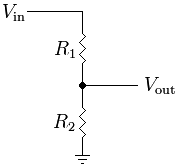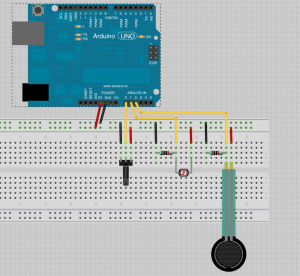# 007 – Voltage Dividers, IR Sensors, Servo Motors

### Voltage Divider

A lot of sensors are basically just resistors that change their resistance based on some external input. The change of resistance can be converted to voltage with a voltage divider.When we read the voltage between two resistors. The balance between the two resistor defines the output voltage.

### Example 1

This example reads the value from three different analog inputs: a potentiometer, a light sensor and a force resistive sensor. The values are just printed out on the serial port.```int potentiometer = 0;
int lightSensor = 0;
int forceSensor = 0;

void setup(){
Serial.begin(9600);
}

void loop(){